# Move the Matches Puzzles Episode 3 Level 41-60 Walkthrough

Walkthrough for all the solutions and answers to the game Move the Matches Puzzles by K-Lab. Get the app on the Google Play Store to play along if you do not already have it.  This is for episode 3, levels 41 through 60.

The images show the final state of the puzzle, so you have to add, move, or remove matches to get the exact picture shown here in this walkthrough.

Enjoy this walkthrough, and let us know what other games you want us to have a walkthrough or review for!

###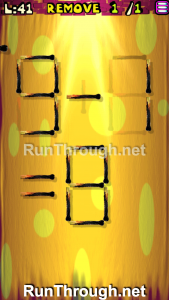Instructions: Remove 1 match to correct the equation

Solution: 9-1=8

###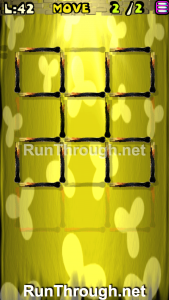Instructions: Move 2 matches to get 5 squares, all equal

###Instructions: Remove 1 match to correct the equation

Solution: 2+9=11

###Instructions: Add 2 matches to correct the equation

Solution: 8-8=0

###Instructions: Move 2 matches to get 3 squares

###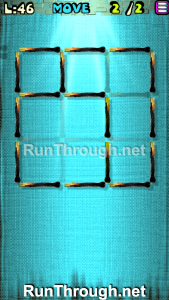Instructions: Move 2 matches to get 3 squares

###Instructions: Remove 2 matches to correct the equation

Solution: 5+6=11

###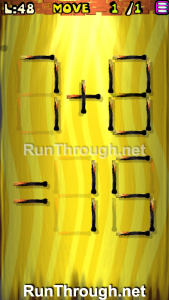Instructions: Move 1 match to correct the equation

Solution: 7+8=15

###Instructions: Remove 2 matches to correct the equation

Solution: 9-4=5

###Instructions: Move 3 matches to get 3 squares

###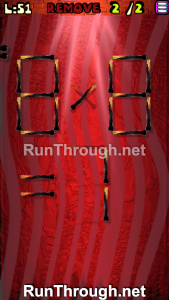Instructions: Remove 2 matches to correct the equation

Solution: 8/8=1

###Instructions: Move 1 match to correct the equation

Solution: 6+7=13

###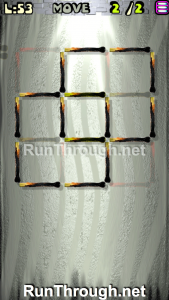Instructions: Move 2 matches to get 3 squares

###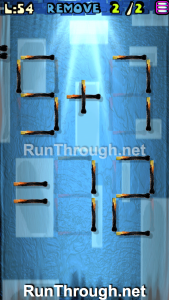Instructions: Remove 2 matches to correct the equation

Solution: 5+7=12

###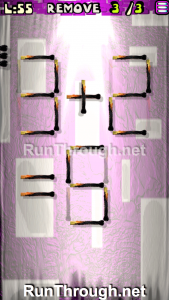Instructions: Remove 3 matches to correct the equation

Solution: 3+2=5

###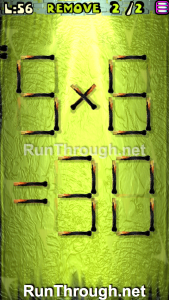Instructions: Remove 2 matches to correct the equation

Solution: 5×6=30

###Instructions: Move 1 match to correct the equation

Solution: 7+8=15

###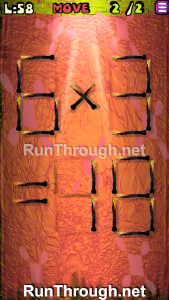Instructions: Move 2 matches to correct the equation

Solution: 6×3=18

###Instructions: Move 3 matches to get 2 triangles

### Level 60Instructions: Move 3 matches to get 4 triangles, all equal